# Beginning Algebra Review Exam 3## Material covered: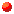Adding and Subtraction Polynomials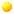Multiplying Polynomials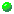Dividing Polynomials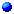Factoring Polynomials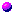Solving Quadratic Equations By Factoring

```I.  Polynomials:  Perform the indicated operations.

A.   1.  (3x² - 5x + 4) + (7x² + 9x - 8)

2.  (7x² - 5x + 3) - (-2x² + 6x - 4)

3.  5x(x - 4) - 3x(2x + 1)

4.  (6x² y)(4x³ y² )

5.  (3x - 8)(4x + 1)

6.  (2x²  + 3x - 1)(x²  + 5x + 4)

7.  (4a³ )²

8.  (5x - 3)²

9.  (5x³ y³ )/(xy² )

10.  (14x³  - 22x)/(2x)

11.  (2x³ + x - 4)/(x - 2)

B.   1.  Subtract x²  + 4x - 3 from 2x²  + 7x - 8.

2.  What is the degree of the polynomial 4x²  + 7x³  - 3x  +  9 ?

II. Factoring.

A.  Completely factor.

1.  3x²  - 9x - 30

2.  6x²  - 7x - 10

3.  50x²  - 32

4.  49x²  - 42x + 9

5.  x²  - 5x  - 36

6.  125x³ - 64

7.  27x³ + 8

B.  Solve for x.

1.  2x²  - 3 = -5x

2.  4x²  - 20x + 25 = 0

3.  5x²  + 6x - 8 = 0

C.  Stated Problems.

1.  The base of a triangle is four feet shorter than twice its altitude.  If the area
of the triangle is 48 square feet, find its dimensions.

2.  The base of a triangle is four feet shorter than twice its altitude.  If the area
of the triangle is 48 square feet, find its dimensions.

```